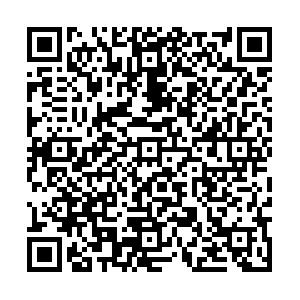## 留言板引用本文: 马丽新，刘晨，刘磊. 基于actor-critic算法的分数阶多自主体系统最优主-从一致性控制 [J]. 应用数学和力学，2022，43（1）：104-114MA Lixin, LIU Chen, LIU Lei. Optimal Leader-Following Consensus Control of Fractional-Order Multi-Agent Systems Based on the Actor-Critic Algorithm[J]. Applied Mathematics and Mechanics, 2022, 43(1): 104-114. doi: 10.21656/1000-0887.420124
 Citation: MA Lixin, LIU Chen, LIU Lei. Optimal Leader-Following Consensus Control of Fractional-Order Multi-Agent Systems Based on the Actor-Critic Algorithm[J]. Applied Mathematics and Mechanics, 2022, 43(1): 104-114.## 基于actor-critic算法的分数阶多自主体系统最优主-从一致性控制

##### doi: 10.21656/1000-0887.420124

###### 作者简介:马丽新（1997—），女，硕士生(E-mail：1623406486@qq.com)刘晨（1993—），男，博士生(E-mail：liuchen_hhu@163.com)刘磊（1983—），男，副教授，博士生导师(通讯作者. E-mail：liulei_hust@163.com)
• 中图分类号: TP273; O232

## Optimal Leader-Following Consensus Control of Fractional-Order Multi-Agent Systems Based on the Actor-Critic Algorithm

• 摘要:

研究了分数阶多自主体系统的最优主-从一致性问题。在考虑控制器周期间歇的前提下，将分数阶微分的一阶近似逼近式、事件触发机制和强化学习中的actor-critic算法有机整合，设计了基于周期间歇事件触发策略的强化学习算法结构。最后，通过数值仿真实验证明了该算法的可行性和有效性。

• 图  1  多自主体系统网络拓扑图(1个领导者，3个追随者)

Figure  1.  The net topology of the multi-agent system (1 leader, 3 followers)

图  2  无控制器作用时，各自主体的状态轨迹(1个领导者，3个追随者)

Figure  2.  State trajectories of each agent without controllers (1 leader, 3 followers)

图  3  各自主体的状态轨迹(1个领导者，3个追随者)

Figure  3.  State trajectories of each agent (1 leader, 3 followers)

图  4  $\|\boldsymbol{e}\left(t\right)\|$及触发阈值变化曲线(1个领导者，3个追随者)

Figure  4.  The error and the trigger threshold (1 leader, 3 followers)

图  5  周期间歇事件触发时刻分布

Figure  5.  The event-trigger moment distribution of periodic intermittence

图  6  多自主体系统网络拓扑图(1个领导者，4个追随者)

Figure  6.  The net topology of the multi-agent system (1 leader, 4 followers)

图  7  无控制器作用时，各自主体的状态轨迹(1个领导者，4个追随者)

Figure  7.  State trajectories of each agent without controllers (1 leader, 4 followers)

图  8  各自主体的状态轨迹(1个领导者，4个追随者)

Figure  8.  State trajectories of each agent (1 leader, 4 followers)

图  9  $\left|\left|{{\boldsymbol{e}}}\left(t\right)\right|\right|$及触发阈值变化曲线(1个领导者，4个追随者)

Figure  9.  The error and the trigger threshold (1 leader, 4 followers)

图  10  事件触发时刻分布

Figure  10.  The event-trigger moment distribution

图  11  文献控制器下，各自主体的状态轨迹图

Figure  11.  State trajectories of each agent under ref. 

图  12  $\left|\left|{{\boldsymbol{e}}}\left(t\right)\right|\right|$及触发阈值变化曲线

Figure  12.  The error $\left|\left|{{\boldsymbol{e}}}\left(t\right)\right|\right|$and the trigger threshold

图  13  事件触发时刻分布

Figure  13.  The event-trigger moment distribution

表  1  网络参数设置

Table  1.   Values of networks’ parameters

 parameter meaning value ${\beta }_{{\rm{c}}1}$ learning rate of the critic network 0.1 ${\beta }_{{\rm{a}}1}$ learning rate of the actor network 0.1 ${T}_{{\rm{c}},\mathrm{e}\mathrm{r}\mathrm{r}\mathrm{o}\mathrm{r} }$ threshold for the critic network ${10^{ - 10}}$ ${T}_{{\rm{a}},\mathrm{e}\mathrm{r}\mathrm{r}\mathrm{o}\mathrm{r} }$ threshold for the actor network ${10^{ - 10}}$ ${N}_{{\rm{c}}1}$ number of hidden nodes in the critic network 5 ${N}_{{\rm{a}}1}$ number of hidden nodes in the critic network 3 ${\psi }_{{\rm{c}}}\left(\cdot \right)$ activation function of the critic network $\mathrm{tan}\mathrm{h}\left(\cdot \right)$ ${\psi }_{{\rm{a}}}\left(\cdot \right)$ activation function of the actor network $\mathrm{tan}\mathrm{h}\left(\cdot \right)$
•点击查看大图
##### 计量
• 文章访问数:  356
• HTML全文浏览量:  172
• PDF下载量:  38
• 被引次数: 0
##### 出版历程
• 收稿日期:  2021-05-07
• 录用日期:  2021-05-07
• 修回日期:  2021-12-03
• 网络出版日期:  2021-12-17
• 刊出日期:  2022-01-01

### 目录/下载:  全尺寸图片 幻灯片
• 分享
• 用微信扫码二维码

分享至好友和朋友圈